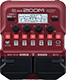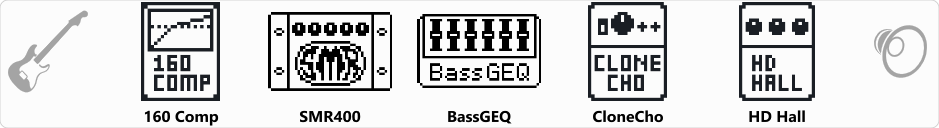# H.A.R.M reverb bass

Discussion in 'Zoom B1/B1X Four' started by alejandro rodas martinez, Aug 1, 2022.

1. ### alejandro rodas martinezNew Member

H.A.R.M reverb bassDevice: Zoom B1 Four
Firmware: 2.00

Name on device: H.bsreveb
Optimized for: Phones/Speaker

Effects chain:poco efectopero ideal para dar espacialidad.. low fx but great to create spatial sensation

Effect: "160 Comp" (Dynamics), active - "yes"
"Threshold" = -15
"Ratio" = 3.7
"Knee" = HARD
"Volume" = 61

Effect: "SMR400" (Amp simulator), active - "yes"
"Bass" = 7.5
"MID-F" = 250Hz
"Middle" = 5.0
"Treble" = 5.0
"Gain" = 59
"ENHNC" = 51
"Volume" = 62

Effect: "BassGEQ" (Filter), active - "yes"
"50" = 4.0
"120" = 3.5
"400" = 3.5
"500" = 3.5
"800" = 3.5
"4.5k" = 2.0
"10k" = 0.0
"VOL" = 81

Effect: "CloneCho" (Modulation), active - "yes"
"Depth" = 2
"Rate" = 8
"Tone" = 85
"Mix" = 53

Effect: "HD Hall" (Reverb), active - "yes"
"PreD" = 81
"Decay" = 45
"Mix" = 44
"Tail" = On

Patch Volume: 66